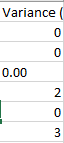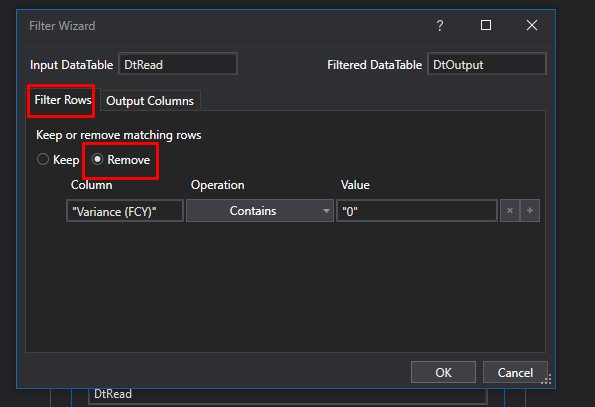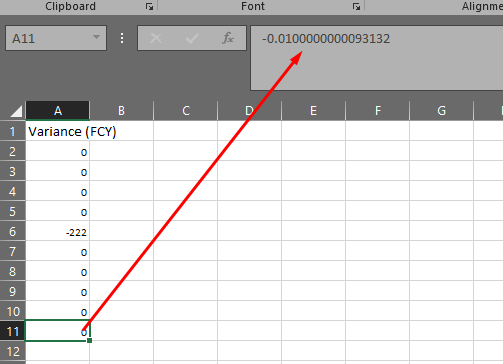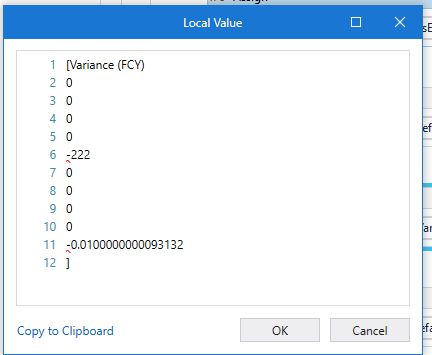# How to filter out the zero value from that column?

Hello Good People,

Am trying to filter out 0 for that column using the linq query below but it isn’t working and I’ve tried others but I have failed.``````dTVariances.AsEnumerable.Where(Function(row) Not double.Parse(row("Variance (FCY)").ToString)=0).CopyToDataTable
``````
``````dTVariances.AsEnumerable().Where(Function(row) Information.IsNumeric(row(8).ToString)).AsEnumerable().Where(function(row) Not double.Parse(row(8).ToString)=0).CopyToDataTable
``````

Kindly advise on what I might be missing out on.
Thank you.

Kakooza Allan Klaus

Hello @Kakooza-Allan-Klaus,

Kindly try this assign.

dtVariances.AsEnumerable.Where(Function (row) not row("Variance (FCY) ").toString = “0” or not row("Variance (FCY) ").toString = “0.00”).CopyToDatatable

Kind Regards.

dTVariances.AsEnumerable.Where(Function(row) Not (Double.Parse(row(“Variance (FCY)”).ToString)=0 Orelse Double.Parse(row(“Variance (FCY)”).ToString)=0.00)).CopyToDataTable

Hello @Kakooza-Allan-Klaus

You can use Filter datatable activity? Add the condition as that column starting with “0”.

Thanks

Still getting the wrong output

Your datatable should consists of rows =0 right not 0.00

Regards
Sudharsan

Can you share the sample input excel file ?

You can also try with Filter DataTable activity

Regards
Gokul

Both of them
@Sudharsan_Ka

Okay Try with this @Kakooza-Allan-Klaus

``````dT.AsEnumerable().Where(Function(row) Information.IsNumeric(row(0).ToString)).AsEnumerable().Where(function(row) Cint(row(0).ToString)=0).CopyToDataTable
``````

Regards
Sudharsan

@Kakooza-Allan-Klaus Use the above expression to get the rows which contains 0 or 0.00

If you dont want those rows contains 0 try with the below expression

``````dT.AsEnumerable().Where(Function(row) Information.IsNumeric(row(0).ToString)).AsEnumerable().Where(function(row) Cdbl(row(0).ToString)<>0).CopyToDataTable
``````

Regards
Sudharsan

@Sudharsan_Ka @Gokul001
I’ve no idea why this query isn’t working because to me everything seems to be fine.
Input.xlsx (9.3 KB)
I’ve shared the input file

Hello @Kakooza-Allan-Klaus,

This works for me, can you kindly try?

dtVariances.AsEnumerable.Where(Function (x) not x(“Variance(FCY)”).toString.trim.Equals(“0”)).CopyToDatatable

You can try with Filter DataTable activity

Check out the XAML file

FilterDatatable_1.xaml (6.5 KB)Regards
Gokul

What count have you got when you tried the input you sent here? @Kakooza-Allan-Klaus

Did you try it out with the above input file because it has failed to work from my end
Am really confused on what’s happening here

Yes, i tried with your input. My output is only -222 and last row. Because last row is not 0, it is -0.0100000000093132.

I think so becasue of this decimel you will get 2 non zero count and 8 zero count @Kakooza-Allan-KlausRegards
Sudharsan

Am aware of that but still get wrong outputFirst What output do you expect? @Kakooza-Allan-Klaus

If you dont want that zero with the decimal to your datatable try with this @Kakooza-Allan-Klaus

``````dT.AsEnumerable().Where(Function(row) Information.IsNumeric(row(0).ToString)).AsEnumerable().Where(function(row) Cint(row(0).ToString)<>0).CopyToDataTable
``````

Regards
Sudharsan